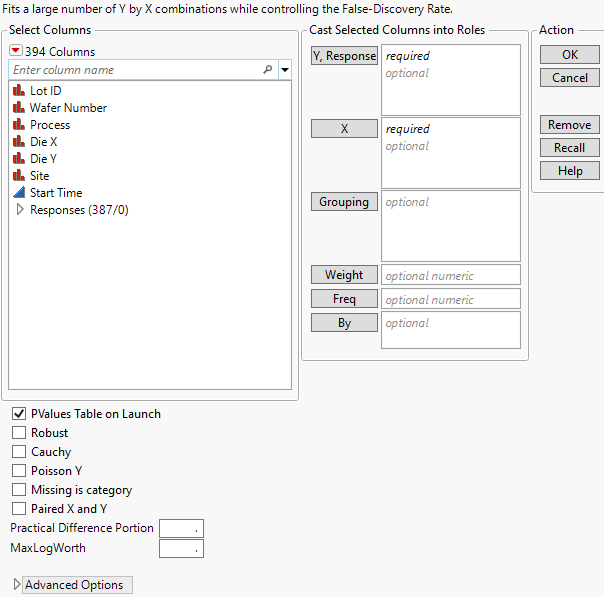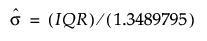Predictive and Specialized Modeling > Response Screening > Launch the Response Screening Platform
Publication date: 11/10/2021

# Launch the Response Screening Platform

Launch the Response Screening platform by selecting Analyze > Screening > Response Screening.

Figure 22.3 Response Screening Launch Window#### Launch Window Roles

Y, Response

Identifies the response columns containing the measurements to be analyzed.

X

Identifies the columns against which you want to test the responses.

Grouping

Analyzes the rows assigned to each level of the specified column separately. All results are presented in a single table and report.

Weight

Identifies a column whose values assign a weight to each row. These values are used as weights in the analysis. See Weight in Fitting Linear Models.

Freq

Identifies a column whose values assign a frequency to each row. These values enable you to account for pre-summarized data. See Frequency in Fitting Linear Models.

By

For each level of the specified column, analyzes the corresponding Ys and Xs and presents the results in separate tables and reports.

#### Launch Window Options

PValues Table on Launch

Creates a data table for the p-values and individual model fit statistics. This data table is linked to the Result Table in the Response Screening report.

Robust

(Available only for continuous responses.) Fits regression and ANOVA models using the Huber M-estimation method (Huber and Ronchetti 2009). This method estimates parameters by minimizing the Huber loss function. The Huber loss function penalizes outliers, which reduces the influence of the outliers on tests. If there are no outliers, the estimates are close to the least squares estimates. When you select the Robust option, several Robust measures and model fit statistics columns are added to the Result Table. See Fit Robust in Basic Analysis for more information about Huber M-estimation. For an example, see Example of Robust Fit.

Caution: The Robust option can increase processing time.

Cauchy

(Available only for continuous responses.) Estimates parameters using maximum likelihood and a Cauchy link function. This estimation method assumes that the errors have a Cauchy distribution, which has fatter tails than the normal distribution, resulting in a reduced emphasis on outliers. This option can be useful if you have a large proportion of outliers in your data. However, if your data are close to normal with only a few outliers, this option can lead to incorrect inferences. When you select the Cauchy option, several Cauchy measures and model fit statistics columns are added to the Result Table.

Poisson Y

Fits each Y response as a count having a Poisson distribution. The test is performed only for categorical X. This option is appropriate when your responses are counts.

Missing is category

For any categorical X variable, treats missing values on X as a category.

Paired X and Y

Performs tests only for Y columns paired with X columns according to their order in the Y, Response and X lists. The first Y is paired with the first X, the second Y with the second X, and so on.

Practical Difference Portion

The fraction of the specification range, or of an estimated six standard deviation range, that represents a difference that you consider pragmatically meaningful. If Spec Limits is not set as a column property, a range of six standard deviations is estimated for the response. The standard deviation estimate is computed from the interquartile range (IQR), as.

If no Practical Difference Proportion is specified, its value defaults to 0.10. Tests of practical significance and equivalence tests use this difference to determine the practical difference. See Compare Means Data Table.

MaxLogWorth

Controls the scale of plots involving LogWorth values (-log10 of p-values). LogWorth values that exceed MaxLogWorth are plotted as MaxLogWorth to prevent extreme scales in LogWorth plots. See Example of the MaxLogWorth Option for an example.

Contains the following options:

Kappa

Adds a new column called Kappa to the data table. If Y and X are both categorical and have the same levels, kappa is provided. This is a measure of agreement between Y and X.

Corr

The Corr option computes the Pearson product-moment correlation in terms of the indices defined by the value ordering.

If X and Y are both binary, the Pearson product-moment calculation gives Spearman’s rho and Kendall's Tau-b. Otherwise, a value of Corr that is large in magnitude indicates an association; a Corr value that is small in magnitude does not preclude an association.

Force X Categorical

Ignores the modeling type and treats all X columns as categorical.

Force X Continuous

Ignores the modeling type and treats all X columns as continuous.

Force Y Categorical

Ignores the modeling type and treats all Y columns as categorical.

Force Y Continuous

Ignores the modeling type and treats all Y columns as continuous.